# Sparse structure estimation for multivariate count data with PLN-network

## Preliminaries

This vignette illustrates the standard use of the PLNnetwork function and the methods accompanying the R6 Classes PLNnetworkfamily and PLNnetworkfit.

### Requirements

The packages required for the analysis are PLNmodels plus some others for data manipulation and representation:

library(PLNmodels)
library(ggplot2)

### Data set

We illustrate our point with the trichoptera data set, a full description of which can be found in the corresponding vignette. Data preparation is also detailed in the specific vignette.

data(trichoptera)
trichoptera <- prepare_data(trichoptera$Abundance, trichoptera$Covariate)

The trichoptera data frame stores a matrix of counts (trichoptera$Abundance), a matrix of offsets (trichoptera$Offset) and some vectors of covariates (trichoptera$Wind, trichoptera$Temperature, etc.)

### Mathematical background

The network model for multivariate count data that we introduce in Chiquet, Robin, and Mariadassou (2019) is a variant of the Poisson Lognormal model of Aitchison and Ho (1989), see the PLN vignette as a reminder. Compare to the standard PLN model we add a sparsity constraint on the inverse covariance matrix $${\boldsymbol\Sigma}^{-1}\triangleq \boldsymbol\Omega$$ by means of the $$\ell_1$$-norm, such that $$\|\boldsymbol\Omega\|_1 < c$$. PLN-network is the equivalent of the sparse multivariate Gaussian model (Banerjee, Ghaoui, and d’Aspremont 2008) in the PLN framework. It relates some $$p$$-dimensional observation vectors $$\mathbf{Y}_i$$ to some $$p$$-dimensional vectors of Gaussian latent variables $$\mathbf{Z}_i$$ as follows $\begin{equation} \begin{array}{rcl} \text{latent space } & \mathbf{Z}_i \sim \mathcal{N}\left({\boldsymbol\mu},\boldsymbol\Omega^{-1}\right) & \|\boldsymbol\Omega\|_1 < c \\ \text{observation space } & Y_{ij} | Z_{ij} \quad \text{indep.} & Y_{ij} | Z_{ij} \sim \mathcal{P}\left(\exp\{Z_{ij}\}\right) \end{array} \end{equation}$

The parameter $${\boldsymbol\mu}$$ corresponds to the main effects and the latent covariance matrix $$\boldsymbol\Sigma$$ describes the underlying structure of dependence between the $$p$$ variables.

The $$\ell_1$$-penalty on $$\boldsymbol\Omega$$ induces sparsity and selection of important direct relationships between entities. Hence, the support of $$\boldsymbol\Omega$$ correspond to a network of underlying interactions. The sparsity level ($$c$$ in the above mathematical model), which corresponds to the number of edges in the network, is controlled by a penalty parameter in the optimization process sometimes referred to as $$\lambda$$. All mathematical details can be found in Chiquet, Robin, and Mariadassou (2019).

#### Covariates and offsets

Just like PLN, PLN-network generalizes to a formulation close to a multivariate generalized linear model where the main effect is due to a linear combination of $$d$$ covariates $$\mathbf{x}_i$$ and to a vector $$\mathbf{o}_i$$ of $$p$$ offsets in sample $$i$$. The latent layer then reads $\begin{equation} \mathbf{Z}_i \sim \mathcal{N}\left({\mathbf{o}_i + \mathbf{x}_i^\top\boldsymbol\Theta},\boldsymbol\Omega^{-1}\right), \qquad \|\boldsymbol\Omega\|_1 < c , \end{equation}$ where $$\boldsymbol\Theta$$ is a $$d\times p$$ matrix of regression parameters.

#### Alternating optimization

Regularization via sparsification of $$\boldsymbol\Omega$$ and visualization of the consecutive network is the main objective in PLN-network. To reach this goal, we need to first estimate the model parameters. Inference in PLN-network focuses on the regression parameters $$\boldsymbol\Theta$$ and the inverse covariance $$\boldsymbol\Omega$$. Technically speaking, we adopt a variational strategy to approximate the $$\ell_1$$-penalized log-likelihood function and optimize the consecutive sparse variational surrogate with an optimization scheme that alternates between two step

1. a gradient-ascent-step, performed with the CCSA algorithm of Svanberg (2002) implemented in the C++ library (Johnson 2011), which we link to the package.
2. a penalized log-likelihood step, performed with the graphical-Lasso of Friedman, Hastie, and Tibshirani (2008), implemented in the package fastglasso (Sustik and Calderhead 2012).

More technical details can be found in Chiquet, Robin, and Mariadassou (2019)

## Analysis of trichoptera data with a PLNnetwork model

In the package, the sparse PLN-network model is adjusted with the function PLNnetwork, which we review in this section. This function adjusts the model for a series of value of the penalty parameter controlling the number of edges in the network. It then provides a collection of objects with class PLNnetworkfit, corresponding to networks with different levels of density, all stored in an object with class PLNnetworkfamily.

### Adjusting a collection of network - a.k.a. a regularization path

PLNnetwork finds an hopefully appropriate set of penalties on its own. This set can be controlled by the user, but use it with care and check details in ?PLNnetwork. The collection of models is fitted as follows:

network_models <- PLNnetwork(Abundance ~ 1 + offset(log(Offset)), data = trichoptera)
##
##  Initialization...
##  Adjusting 30 PLN with sparse inverse covariance estimation
##  Joint optimization alternating gradient descent and graphical-lasso
##  sparsifying penalty = 3.577926
sparsifying penalty = 3.304826
sparsifying penalty = 3.052572
sparsifying penalty = 2.819571
sparsifying penalty = 2.604356
sparsifying penalty = 2.405568
sparsifying penalty = 2.221953
sparsifying penalty = 2.052353
sparsifying penalty = 1.895699
sparsifying penalty = 1.751002
sparsifying penalty = 1.617349
sparsifying penalty = 1.493898
sparsifying penalty = 1.37987
sparsifying penalty = 1.274546
sparsifying penalty = 1.177261
sparsifying penalty = 1.087402
sparsifying penalty = 1.004401
sparsifying penalty = 0.9277361
sparsifying penalty = 0.8569228
sparsifying penalty = 0.7915146
sparsifying penalty = 0.731099
sparsifying penalty = 0.6752948
sparsifying penalty = 0.6237502
sparsifying penalty = 0.5761399
sparsifying penalty = 0.5321636
sparsifying penalty = 0.491544
sparsifying penalty = 0.4540249
sparsifying penalty = 0.4193695
sparsifying penalty = 0.3873594
sparsifying penalty = 0.3577926
##  Post-treatments
##  DONE!

Note the use of the formula object to specify the model, similar to the one used in the function PLN.

### Structure of PLNnetworkfamily

The network_models variable is an R6 object with class PLNnetworkfamily, which comes with a couple of methods. The most basic is the show/print method, which sends a very basic summary of the estimation process:

network_models
## --------------------------------------------------------
## COLLECTION OF 30 POISSON LOGNORMAL MODELS
## --------------------------------------------------------
## ========================================================
##  - 30 penalties considered: from 0.3577926 to 3.577926
##  - Best model (greater BIC): lambda = 1.38
##  - Best model (greater EBIC): lambda = 1.38

One can also easily access the successive values of the criteria in the collection

network_models$criteria %>% head() %>% knitr::kable() param nb_param loglik BIC ICL R_squared n_edges EBIC pen_loglik density stability 3.577926 17 -2403.074 -2436.154 -3903.033 0.9761923 0 -2436.154 -2409.325 0.0000000 NA 3.304826 17 -2397.653 -2430.734 -3897.580 0.9760751 0 -2430.734 -2403.634 0.0000000 NA 3.052571 17 -2392.513 -2425.593 -3892.439 0.9760751 0 -2425.593 -2398.239 0.0000000 NA 2.819571 18 -2387.654 -2422.680 -3889.526 0.9760751 1 -2425.136 -2393.133 0.0069204 NA 2.604356 18 -2382.908 -2417.934 -3884.780 0.9760751 1 -2420.390 -2388.155 0.0069204 NA 2.405568 18 -2378.465 -2413.491 -3880.337 0.9760751 1 -2415.947 -2383.486 0.0069204 NA A diagnostic of the optimization process is available via the convergence field: network_models$convergence %>% head() %>% knitr::kable()
param nb_param objective convergence outer_iterations inner_iterations inner_status inner_message
3.577926 17 2409.32513504089 7.37116634980569e-06 15 53 4 xtol_rel or xtol_abs was reached
3.304826 17 2403.63429712207 6.12373179177022e-06 2 29 4 xtol_rel or xtol_abs was reached
3.052571 17 2398.23872142406 3.79234433022946e-16 2 19 4 xtol_rel or xtol_abs was reached
2.819571 18 2393.13330572702 0 2 17 4 xtol_rel or xtol_abs was reached
2.604356 18 2388.15519041443 0 2 17 4 xtol_rel or xtol_abs was reached
2.405568 18 2383.48593572793 0 2 17 4 xtol_rel or xtol_abs was reached

An nicer view of this output comes with the option “diagnostic” in the plot method:

plot(network_models, "diagnostic")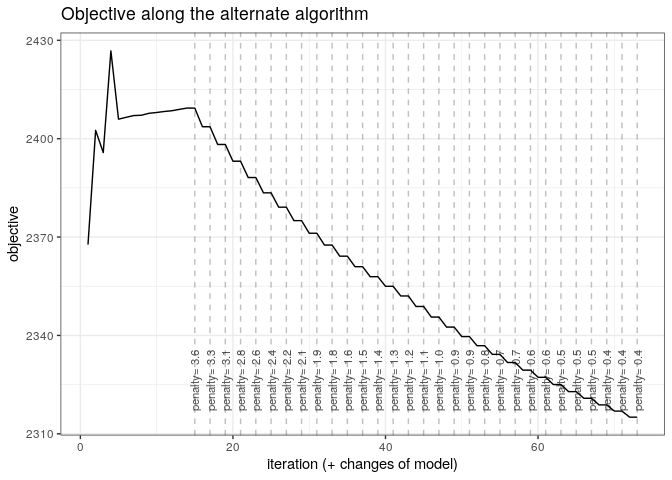### Exploring the path of networks

By default, the plot method of PLNnetworkfamily displays evolution of the criteria mentioned above, and is a good starting point for model selection:

plot(network_models)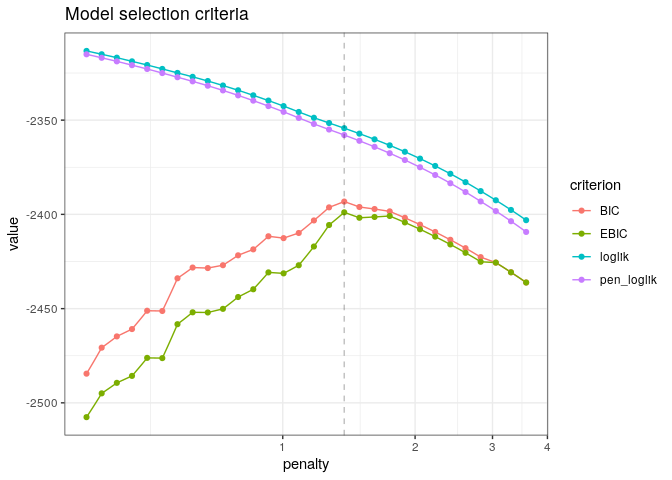In this case, the variational lower bound of the log-likelihood is hopefully strictly increasing with a lower level of penalty (meaning more edges in the network). The same holds true for the penalized counterpart of the variational surrogate. Generally, smoothness of these criteria is a good sanity check of optimization process. BIC and its extended-version high-dimensional version EBIC are classically used for selecting the correct amount of penalization with sparse estimator like the one used by PLN-network. However, we will consider later a more robust albeit more computationally intensive strategy to chose the appropriate number of edges in the network.

To pursue the analysis, we can represent the coefficient path (i.e., value of the edges in the network according to the penalty level) to see if some edges clearly come off. An alternative and more intuitive view consists in plotting the values of the partial correlations along the path, which can be obtained with the options corr = TRUE. To this end, we provide the S3 function coefficient_path:

coefficient_path(network_models, corr = TRUE) %>%
ggplot(aes(x = Penalty, y = Coeff, group = Edge, colour = Edge)) +
geom_line(show.legend = FALSE) +  coord_trans(x="log10") + theme_bw()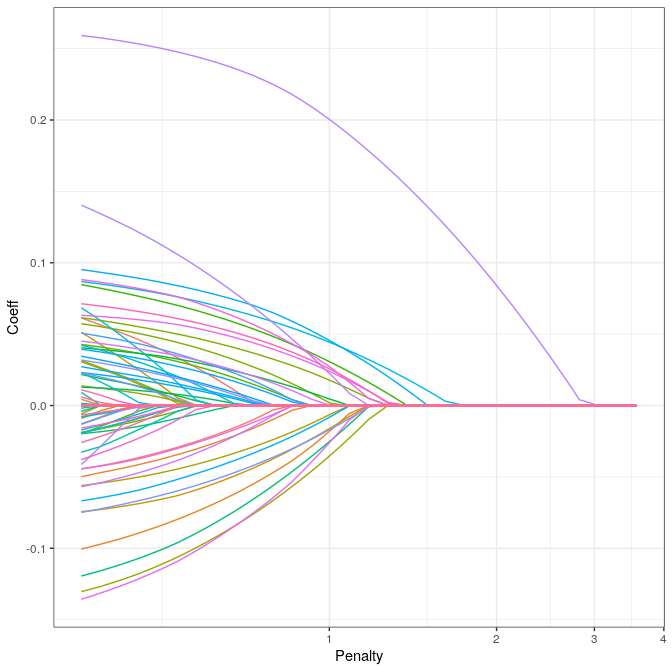### Model selection issue: choosing a network

To select a network with a specific level of penalty, one uses the getModel(lambda) S3 method. We can also extract the best model according to the BIC or EBIC with the method getBestModel().

model_pen <- getModel(network_models, network_models$penalties) # give some sparsity model_BIC <- getBestModel(network_models, "BIC") # if no criteria is specified, the best BIC is used An alternative strategy is to use StARS (Liu, Roeder, and Wasserman 2010), which performs resampling to evaluate the robustness of the network along the path of solutions in a similar fashion as the stability selection approach of Meinshausen and Bühlmann (2010), but in a network inference context. model_StARS <- getBestModel(network_models, "StARS") # if StARS is requested, stability selection is performed if needed  ## ## Stability Selection for PLNnetwork: ## subsampling: ++++++++++++++++++++ When “StARS” is requested for the first time, getBestModel automatically calls the method stability_selection with the default parameters. After the first call, the stability path is available from the plot function: plot(network_models, "stability")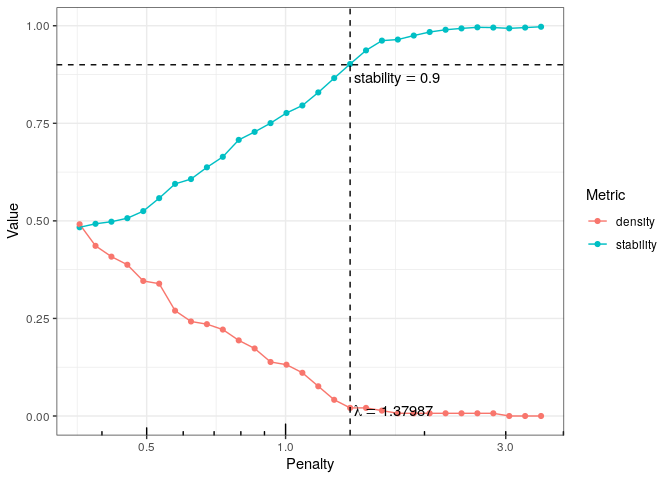### Structure of a PLNnetworkfit The variables model_BIC, model_StARS and model_pen are other R6Class objects with class PLNnetworkfit. They all inherits from the class PLNfit and thus own all its methods, with a couple of specific one, mostly for network visualization purposes. Most fields and methods are recalled when such an object is printed: model_StARS ## Poisson Lognormal with sparse inverse covariance (penalty = 1.38) ## ================================================================== ## nb_param loglik BIC ICL R_squared n_edges EBIC pen_loglik ## 20 -2354.283 -2393.201 -3860.047 0.976 3 -2398.922 -2357.905 ## density ## 0.021 ## ================================================================== ## * Useful fields ##$model_par, $latent,$var_par, $optim_par ##$loglik, $BIC,$ICL, $loglik_vec,$nb_param, $criteria ## * Useful S3 methods ## print(), coef(), sigma(), vcov(), fitted(), predict(), standard_error() ## * Additional fields for sparse network ##$EBIC, $density,$penalty
## * Additional S3 methods for network
##     plot.PLNnetworkfit()

The plot method provides a quick representation of the inferred network, with various options (either as a matrix, a graph, and always send back the plotted object invisibly if users needs to perform additional analyses).

my_graph <- plot(model_StARS, plot = FALSE)
my_graph
## IGRAPH db67602 UNW- 17 3 --
## + attr: name (v/c), label (v/c), label.cex (v/n), size (v/n),
## | label.color (v/c), frame.color (v/l), weight (e/n), color (e/c),
## | width (e/n)
## + edges from db67602 (vertex names):
##  Che--Hve Glo--Hfo Hfo--Hsp
plot(model_StARS)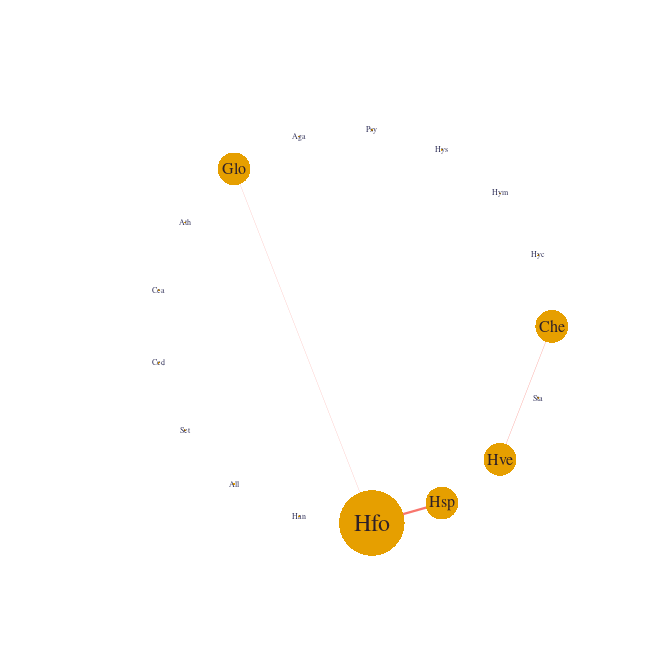plot(model_StARS, type = "support", output = "corrplot")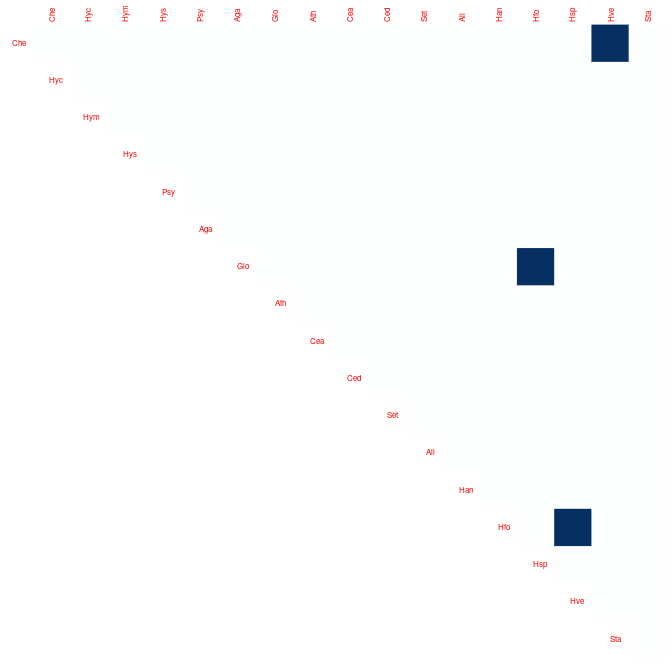We can finally check that the fitted value of the counts – even with sparse regularization of the covariance matrix – are close to the observed ones:

data.frame(
fitted   = as.vector(fitted(model_StARS)),
observed = as.vector(trichoptera\$Abundance)
) %>%
ggplot(aes(x = observed, y = fitted)) +
geom_point(size = .5, alpha =.25 ) +
scale_x_log10(limits = c(1,1000)) +
scale_y_log10(limits = c(1,1000)) +
theme_bw() + annotation_logticks()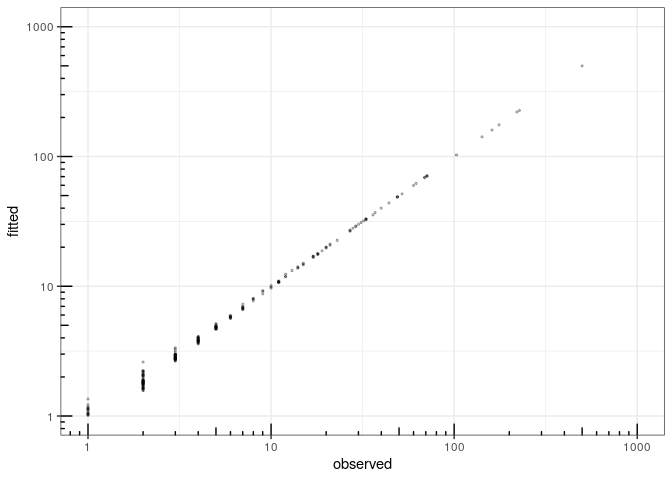fitted value vs. observation